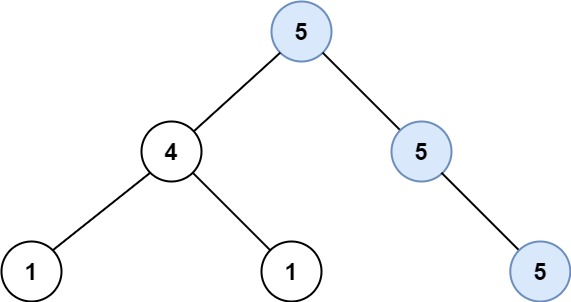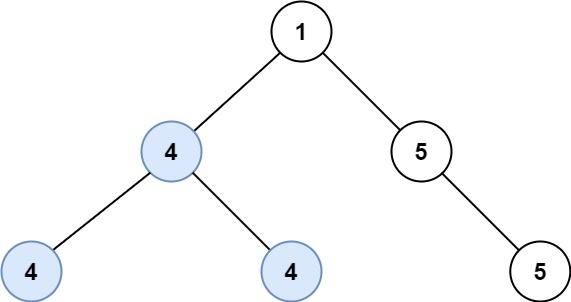# LeetCode: 687. Longest Univalue Path

## 题目¶

Given the root of a binary tree, return the length of the longest path, where each node in the path has the same value. This path may or may not pass through the root.

The length of the path between two nodes is represented by the number of edges between them.

Example 1:```Input: root = [5,4,5,1,1,5]
Output: 2
```

Example 2:```Input: root = [1,4,5,4,4,5]
Output: 2
```

Constraints:

• The number of nodes in the tree is in the range [0, 10^4] .
• -1000 <= Node.val <= 1000
• The depth of the tree will not exceed 1000.

## 解法¶

```# Definition for a binary tree node.
# class TreeNode(object):
#     def __init__(self, val=0, left=None, right=None):
#         self.val = val
#         self.left = left
#         self.right = right
class Solution(object):
def longestUnivaluePath(self, root):
self._max_length = 0
self._max_deepth(root)

return self._max_length

def _max_deepth(self, root):
if root is None:
return 0

left_deepth = self._max_deepth(root.left)
right_deepth = self._max_deepth(root.right)

# 如果值不同的话，深度就是 0
if root.left is not None and root.left.val != root.val:
left_deepth = 0
if root.right is not None and root.right.val != root.val:
right_deepth = 0

length = left_deepth + right_deepth
if length > self._max_length:
self._max_length = length

return 1 + max(left_deepth, right_deepth)
```